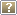## Which is better: Fixed or adjustable-rate mortgage?It is a difficult decision to decide between a fixed and an adjustable-rate mortgage. Factors such as loan duration, the index used by the lender, the number and timing of rate adjustments, and your assumption about the increase/decrease of future interest rates all have an impact. Use this calculator to help compare the total cost of each alternative.
Mortgage Alternatives and Assumptions
Loan amount (\$)
 Fixed-Rate Loan Adjustable-Rate Loan (Initial) annual interest rate Number of years
Index Rate Detail
Current index rate (%)Lenders margin added to index rate (%)Index rate adjustment (%)Number of months between index rate adjustments
What will rates be doing over the life of the loan: stay same, increase or decrease?
Assumed maximum annual rate adjustment (%)
Comparison options/cost of moneyMarginal tax bracket (%)